# Convert Time to Minutes – Excel & Google Sheets

This tutorial will demonstrate how to convert a time value to minutes in Excel & Google Sheets.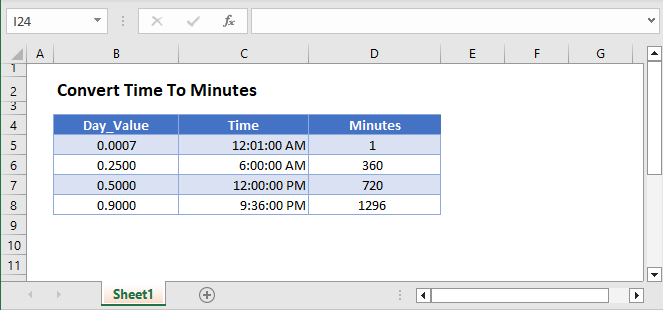## Convert Time to Minutes – Excel

Excel stores time as a decimal value where each 1/24th represents one hour of a day. Understanding this, you can convert a time to minutes by multiplying the time by 1440:

``=B3*1440``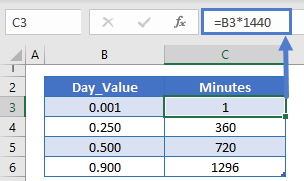Why does this work? Remember that there are 24 hours in a day, 60 minutes in an hour. So there are 24*60=1,440 minutes in a day. By multiplying the time by 1440 you calculate the number of minutes corresponding to the time.

### Format as Number

When you multiply time by 1,440, Excel may format the result as time (ex. h:mm):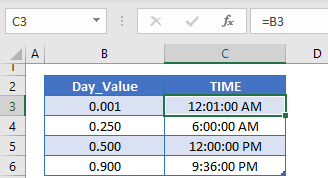To display the result as a regular number, change the formatting to General: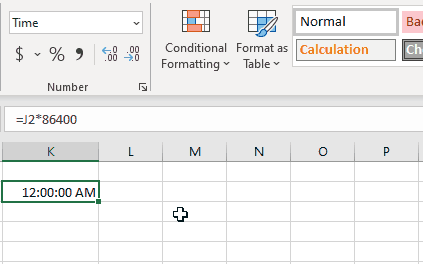## Convert Time to Minutes in Google Sheets

All of the above examples work exactly the same in Google Sheets as in Excel.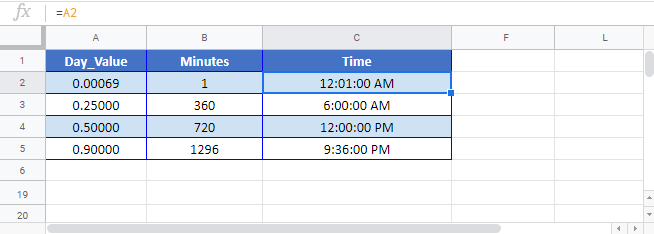### Excel Practice Worksheet

Practice Excel functions and formulas with our 100% free practice worksheets!